Courses

# Notes | EduRev

## NEET : Notes | EduRev

The document Notes | EduRev is a part of the NEET Course Physics 28 Years Past year papers for NEET/AIPMT Class 12.
All you need of NEET at this link: NEET

Q.1. The total energy of an electron in an atom in an orbit is –3.4 eV. Its kinetic and potential energies are, respectively:    (2019)
A: –3.4 eV, –3.4 eV
B: –3.4 eV, –6.8 eV
C: 3.4 eV, –6.8 eV
D: 3.4 eV, 3.4 eV
Ans:
C
Solution:

In Bohr's model of H atom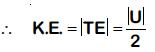∴ K.E. = 3.4 eV
U = –6.8 eV

Q.2. α-particle consists of :    (2019)
A: 2 protons and 2 neutrons only
B: 2 electrons, 2 protons and 2 neutrons
C: 2 electrons and 4 protons only
D: 2 protons only
Ans:
A
Solution:

α-particle is nucleus of Helium which has two protons and two neutrons.

Q.3. The ratio of kinetic energy to the total energy of an electron in a Bohr orbit of the hydrogen atom,is :-    (2018)
A: 1 : 1
B: 1 : –1
C: 2 : –1
D: 1 : –2
Ans:
B
Solution: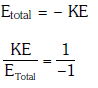Q.4. Suppose the charge of a proton and an electron differ slightly. One of them is – e, the other is(e + De). If the net of electrostatic force and gravitational force between two hydrogen atoms placed at a distance d (much greater than atomic size) apart is zero, then De is of the order of [Given mass of hydrogen mh = 1.67 × 10–27 kg]     (2017)
A: 10–23 C
B: 10–37 C
C: 10–47 C
D: 10–20 C
Ans: B
Solution:

we know, a hydrogen atom has one electron and one proton
so, net charge on each hydrogen atom = (e + ∆e - e) = ∆e
so, electrostatic force ,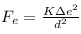gravitational force,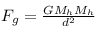where Mh denotes mass of hydrogen
a/c to question,
gravitational force = electrostatic force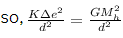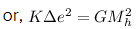we know, K = 9 × 109 Nm²/C², G = 6.67 × 10-11 Nm²/Kg² , Mh = 1.67 × 10-27 Kg
so, 9 × 109 × ∆e² = 6.67 × 10-11 × (1.67 × 10-27)²
or, ∆e² = 6.67 × 10-11 × 1.67 × 1.67 × 10-54/9 × 109
or, ∆e = 1.43767 × 10-37 C

Q.5. The ratio of wavelengths of the last line of Balmer series and the last line of Lyman series is :-     (2017)
A: 1
B: 4
C: 0.5
D: 2
Ans:
B
Solution:

For last line of Balmer : n1 = 2 & n2 = ∞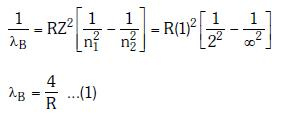For last line Lyman series : n1 = 1 & n2 = ∞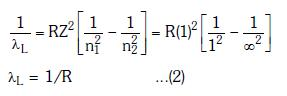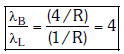Q.6. When an α-particle of mass 'm' moving with velocity 'v' bombards on a heavy nucleus of charge 'Ze' its distance of closet approach from the nucleus depends on m as :    (2016)
A: m
B: 1/m
C: 1/√m
D: 1/m2
Ans: B
Solution:

When an alpha particle moving with velocity v bombards on a heavy nucleus of charge Ze, then there will be no loss of energy.
Initial Kinetic energy of the alpha particle = Potential energy of alpha particle at closest approach.
That is,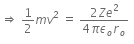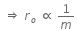This is the required distance of closest approach to alpha particle from the nucleus.
When an alpha particle moving with velocity v bombards on a heavy nucleus of charge Ze, then there will be no loss of energy.
Initial Kinetic energy of the alpha particle = Potential energy of alpha particle at closest approach.
That is,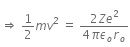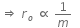This is the required distance of closest approach to alpha particle from the nucleus.

Q.7. Given the value of Rydberg constant is 107 m-1, the wave number of the last line of the Balmer series in hydrogen spectrum will be :     (2016)
A: 2.5 x 107m-1
B: 0.025 x 104m-1
C: 0.5 x 107m-1
D: 0.25 x 107m-1
Ans:
D
Solution:
Rydberg constant, r = 107 m-1
For last line in Balmer series,
n2 = ∞; n1 = 2
We know,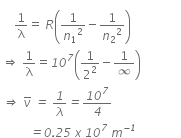Q.8. Consider 3rd orbit of He+ (Helium), using non-relativistic approach, the speed of electron in this orbit will be [given K = 9 x 109 constant, Z = 2 and h(Planck's Constant) = 6.6 x 10-34 J s]    (2015)
A: 3.0 x 108 m/s
B: 2.92 x 106 m/s
C: 1.46 x 106 m/s
D: 0.73 x 106 m/s
Ans: C
Solution: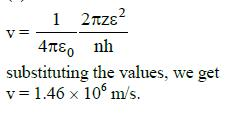Q.9. If radius of the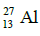nucleus is taken to be RAl, then the radius of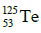nucleus is nearly :    (2015)
A: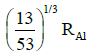B: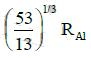C: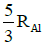D: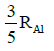Ans:
C
Solution:

Radius of the nucleus goes as R ∝ A1/3, where A is the atomic mass. If RTe is the radius of the nucleus of telurium atom and RAl is the radius of the nucleus of aluminium atom we have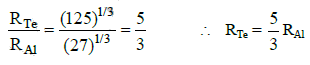Q.10. Hydrogen atom in ground state is excited by a monochromatic radiation of λ = 975 Å. Number of spectral lines in the resulting spectrum emitted will be:     (2014)
A: 6
B: 10
C: 3
D: 2
Ans: A
Solution: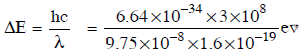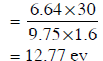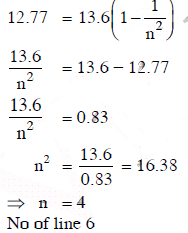Offer running on EduRev: Apply code STAYHOME200 to get INR 200 off on our premium plan EduRev Infinity!

,

,

,

,

,

,

,

,

,

,

,

,

,

,

,

,

,

,

,

,

,

;